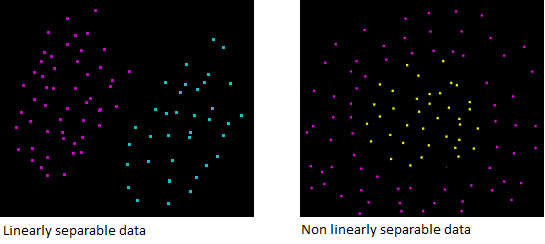## Linear Kernel: Why is it recommended for text classification ?

The Support Vector Machine can be viewed as a kernel machine. As a result, you can change its behavior by using a different kernel function.

The most popular kernel functions are :

• the linear kernel
• the polynomial kernel
• the RBF (Gaussian) kernel
• the string kernel

## The linear kernel is often recommended for text classification

It is interesting to note that :

The original optimal hyperplane algorithm proposed by Vapnik in 1963 was a linear classifier 

That's only 30 years later that the kernel trick was introduced.

If it is the simpler algorithm, why is the linear kernel recommended for text classification?

## Text is often linearly separable

Most of text classification problems are linearly separableLinear kernel works well with linearly separable data

Read more